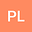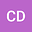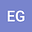Testing machine learning algorithms for the prediction of depositional fluxes of the radionuclides 7Be, 210Pb and 40K.
•••• +1
Pedro De la Torre LuqueIstituto Nazionale di Fisica Nucleare Sezione Bari

Corresponding Author:pedro.delatorreluque@fysik.su.se

Author ProfileConcepcion Duenas
University of Malaga
Author Profile## Abstract

The monthly depositional fluxes of three natural radionuclides ($^{7}$Be, $^{210}$Pb and $^{40}$K) were measured at a Mediterranean coastal station (Malaga, Southern Spain) over a 14-year period from 2005 to 2018, corresponding to 168 monthly samples. The study of these radionuclides provides valuable information on the atmospheric air circulation, transportation and erosion processes as well as a control of the environmental radioactivity. In this work, the depositional fluxes of these radionuclides are investigated and their relations with several atmospheric variables, such as air temperature, pressure or precipitations, have been studied by applying two popular machine learning methods: Random Forest and Neural Network algorithms. We extensively test different configurations of these algorithms and demonstrate their predictive ability for reproducing depositional fluxes of $^{7}$Be, $^{210}$Pb and $^{40}$K. We use the Pearson-R correlation coefficient and the mean average error to evaluate the predictions of the developed models, revealing that the models derived with Neural Networks achieve slightly better results, in average, although similar, having into account the uncertainties. The mean Pearson-R coefficients, evaluated with a k-fold cross-validation method, are around 0.85 for the three radionuclides using Neural Network models, while they go down to 0.83, 0.79 and 0.8 for $^{7}$Be, $^{210}$Pb and $^{40}$K, respectively, for the Random Forest models. Additionally, applying the Recursive Feature Elimination technique we determine the variables more correlated with the depositional fluxes of these radionuclides, which elucidates the main dependencies of their temporal variability.